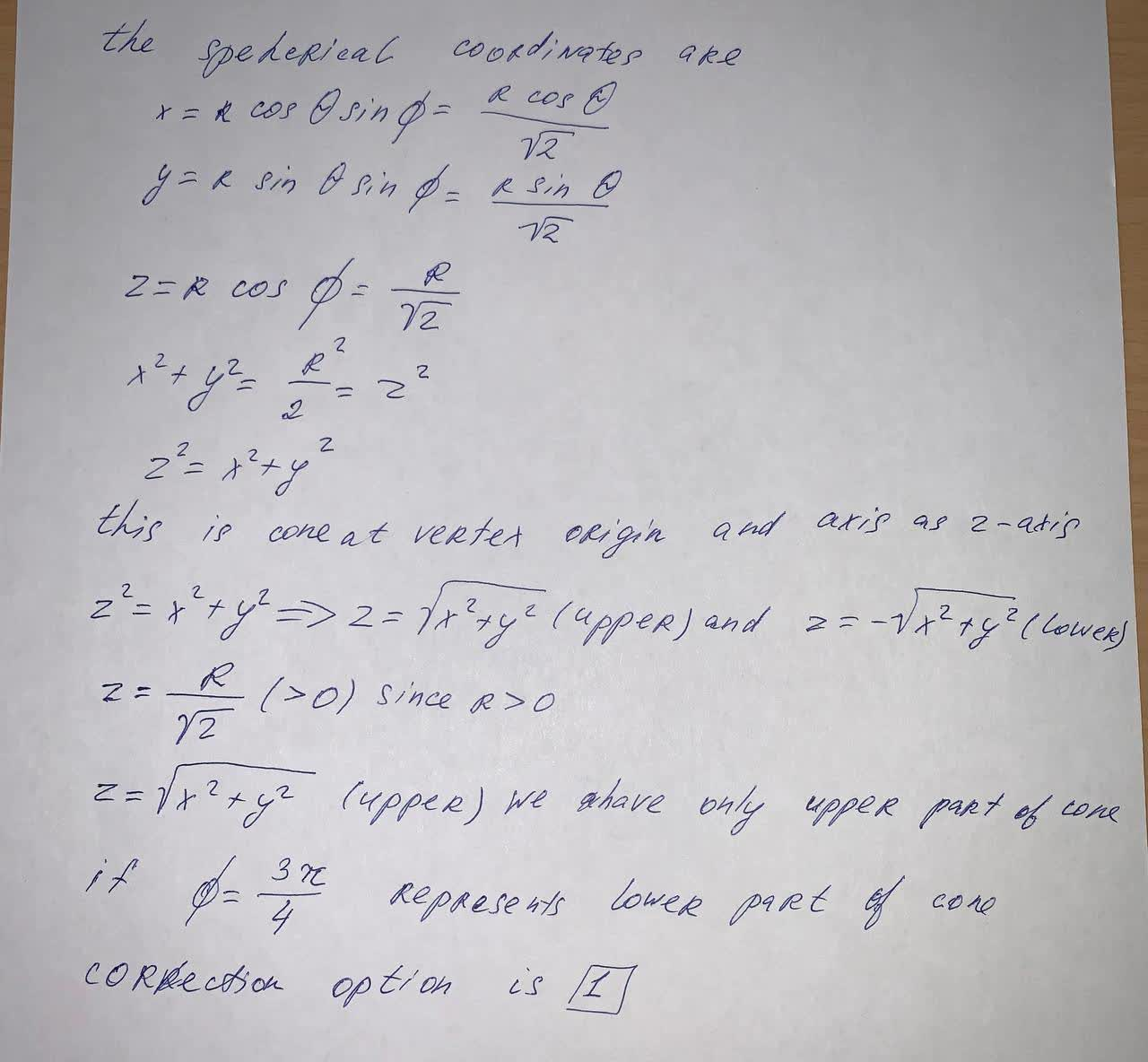# Describe in words the surface whose equation is given. \phi = \frac{\pi}{4} (select the correct answer) 1)the top half of the right circular cone withankarskogC 2021-06-07 Answered
Describe in words the surface whose equation is given. $\varphi =\frac{\pi }{4}$ (select the correct answer)
1)the top half of the right circular cone with vertex at the origin and axis the positive z-axis
2)the plane perpendicular to the xz-plane passing through z = x, where $x\ge 0$
3)the plane perpendicular to the xy-plane passing through y = x, where $x\ge 0$
4)the base of the right circular cone with vertex at the origin and axis the positive z-axis
5)the plane perpendicular to the yz-plane passing through z = y, where $y\ge 0$
You can still ask an expert for help

• Questions are typically answered in as fast as 30 minutes

Solve your problem for the price of one coffee

• Math expert for every subject
• Pay only if we can solve itAyesha Gomez###### Not exactly what you’re looking for?Jeffrey Jordon

The speherial coordinates are

$x=R\mathrm{cos}\theta \mathrm{sin}\varphi =\frac{R\mathrm{cos}\theta }{\sqrt{2}}$

$y=R\mathrm{sin}\theta \mathrm{sin}\varphi =\frac{R\mathrm{sin}\theta }{\sqrt{2}}$

$z=R\mathrm{cos}\varphi =\frac{R}{\sqrt{2}}$

${x}^{2}+{y}^{2}=\frac{{R}^{2}}{2}={z}^{2}$

${z}^{2}={x}^{2}+{y}^{2}$

this is coneat vertex origin and axis as z-axis

${z}^{2}={x}^{2}+{y}^{2}⇒z=\sqrt{{x}^{2}+{y}^{2}}$ (upper) and $z=-\sqrt{{x}^{2}+{y}^{2}}$ (lower)

$z=\sqrt{{x}^{2}+{y}^{2}}$ (upper) we  have only upper part of cone

if $\varphi =\frac{3\pi }{4}$ represents lower part of cone correction option is 1